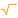# Root

 Block Group: Math Operations Icon:The Root block returns the nth root of a number. The input property must be a non-negative number.

For information on using dataflow blocks, see Dataflow.

For accepted and excluded arguments of Math Operations blocks, see Math Operations.

## Input/Output Properties

The following properties of the Root block can take input and give output.

• input (number)
• root (number)

input specifies the value to take the root of.

root specifies the root to use. For example, a value of 3 calculates a cubic root.

## Output Property

The following property of the Root block can give output but cannot take input.

• output (number)

output returns the result of the calculation.

## Examples

The following image shows four examples of the Root block.